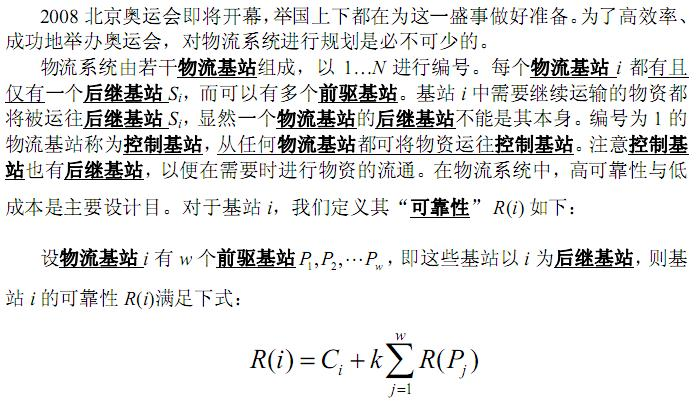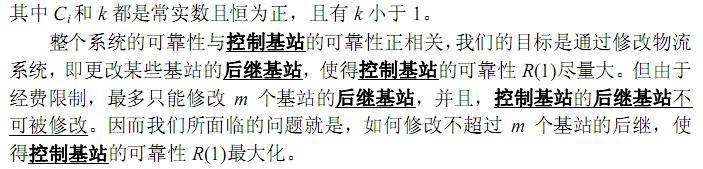「NOI2008」奥运物流 - 树形动规+未来Dp | Bill Yang's Blog

## 题目大意## 题目分析• 不修改$i$的后继，将$j$次修改分配到$i$子树中，再加上当前对$1$的贡献$C_ik^{d(i,1)}$。
• 若修改$i$的后继，假设$d(i,1)=tmp$，那么子树的所有贡献值需要除以$k^{tmp-1}$，但若儿子也修改过，就不能修改其贡献值。

0%